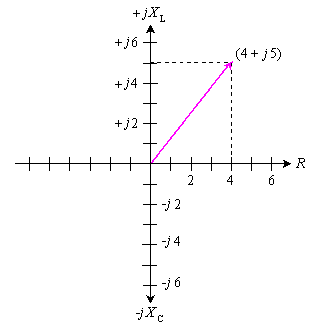Browse Definitions :
Definition

# impedance

## What is impedance?

Impedance, denoted Z, is an expression of the opposition that an electronic component, circuit, or system offers to alternating and/or direct electric current. Impedance is a vector (two-dimensional)quantity consisting of two independent scalar (one-dimensional) phenomena: resistance and reactance.

Resistance, denoted R, is a measure of the extent to which a substance opposes the movement of electrons among its atoms. The more easily the atoms give up and/or accept electrons, the lower the resistance, which is expressed in positive real number ohms. Resistance is observed with alternating current (AC) and also with direct current (DC). Examples of materials with low resistance, known as electrical conductors, include copper, silver, and gold. High-resistance substances are called insulators or dielectrics, and include materials such as polyethylene, mica, and glass. A material with an intermediate levels of resistance is classified as a semiconductor. Examples are silicon, germanium, and gallium arsenide.

Reactance, denoted X, is an expression of the extent to which an electronic component, circuit, or system stores and releases energy as the current and voltage fluctuate with each AC cycle. Reactance is expressed in imaginary number ohms. It is observed for AC, but not for DC. When AC passes through a component that contains reactance, energy might be stored and released in the form of a magnetic field, in which case the reactance is inductive (denoted +jXL); or energy might be stored and released in the form of an electric field, in which case the reactance is capacitive (denoted -jXC). Reactance is conventionally multiplied by the positive square root of -1, which is the unit imaginary number called the j operator, to express Z as a complex number of the form R + jXL (when the net reactance is inductive) or R - jXC (when the net reactance is capacitive).

The illustration shows a coordinate plane modified to denote complex-number impedances. Resistance appears on the horizontal axis, moving toward the right.(The left-hand half of this coordinate plane is not normally used because negative resistances are not encountered in common practice.)Inductive reactance appears on the positive imaginary axis, moving upward. Capacitive reactance is depicted on the negative imaginary axis, moving downward. As an example, a complex impedance consisting of 4 ohms of resistance and +j5 ohms of inductive reactance is denoted as a vector from the origin to the point on the plane corresponding to 4 + j5.In series circuits, resistances and reactances add together independently. Suppose a resistance of 100.00 ohms is connected in a series circuit with an inductance of 10.000 ?H.At 4.0000 MHz, the complex impedance is:

ZRL = R + jXL = 100.00 + j251.33

If a capacitor of 0.0010000 ?F is put in place of the inductor, the resulting complex impedance at 4.0000 MHz is:

ZRC = R - jXC = 100.00 - j39.789

If all three components are connected in series, then the reactances add, yielding a complex impedance of:

ZRLC = 100 + j251.33 - j39.789 = 100 + j211.5

This is the equivalent of a 100-ohm resistor in series with an inductor having +j211.5 ohms of reactance. At 4.0000 MHz, this reactance is presented by an inductance of 8.415 ?H, as determined by plugging the numbers into the formula for inductive reactance and working backwards.(See the definition of for this formula, and for the corresponding formula for capacitive reactance.)

Parallel RLC circuits are more complicated to analyze than are series circuits. To calculate the effects of capacitive and inductive reactance in parallel, the quantities are converted to inductive susceptance and capacitive susceptance. Susceptance is the reciprocal of reactance. Susceptance combines with conductance, which is the reciprocal of resistance, to form complex admittance, which is the reciprocal of complex impedance. Entire volumes have been devoted to the theoretical and practical aspects of resistance, conductance, reactance, susceptance, impedance, and admittance. An intermediate electronics text or reference book is recommended for further study.

This was last updated in December 2021

• User Datagram Protocol (UDP)

User Datagram Protocol (UDP) is a communications protocol primarily used to establish low-latency and loss-tolerating connections...

• Telnet

Telnet is a network protocol used to virtually access a computer and provide a two-way, collaborative and text-based ...

• big-endian and little-endian

The term endianness describes the order in which computer memory stores a sequence of bytes.

An advanced persistent threat (APT) is a prolonged and targeted cyber attack in which an intruder gains access to a network and ...

• Mitre ATT&CK framework

The Mitre ATT&CK (pronounced miter attack) framework is a free, globally accessible knowledge base that describes the latest ...

• timing attack

A timing attack is a type of side-channel attack that exploits the amount of time a computer process runs to gain knowledge about...

• employee resource group (ERG)

An employee resource group is a workplace club or more formally realized affinity group organized around a shared interest or ...

• employee training and development

Employee training and development is a set of activities and programs designed to enhance the knowledge, skills and abilities of ...

• employee sentiment analysis

Employee sentiment analysis is the use of natural language processing and other AI techniques to automatically analyze employee ...

• customer profiling

Customer profiling is the detailed and systematic process of constructing a clear portrait of a company's ideal customer by ...

• customer insight (consumer insight)

Customer insight, also known as consumer insight, is the understanding and interpretation of customer data, behaviors and ...